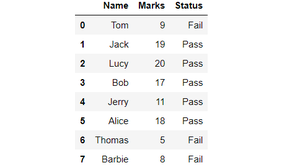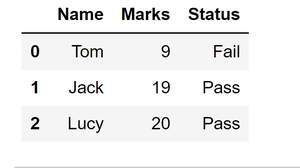# Get the first 3 rows of a given DataFrame

Let us first create a dataframe and then we will try to get first 3 rows of this dataframe using several methods.

Code: Creating a Dataframe.

## Python3

 `# import pandas library ` `import` `pandas as pd ` ` `  `# dictionary ` `record ``=` `{ ` `  ``"Name"``: [``"Tom"``, ``"Jack"``, ``"Lucy"``, ` `           ``"Bob"``, ``"Jerry"``, ``"Alice"``, ` `           ``"Thomas"``, ``"Barbie"``], ` `   `  `   ``"Marks"``: [``9``, ``19``, ``20``,  ` `             ``17``, ``11``, ``18``, ` `             ``5``, ``8``],  ` `   `  `  ``"Status"``: [``"Fail"``, ``"Pass"``, ``"Pass"``, ` `             ``"Pass"``,``"Pass"``, ``"Pass"``, ` `             ``"Fail"``, ``"Fail"``]} ` ` `  `# converting record into ` `# pandas dataframe ` `df ``=` `pd.DataFrame(record) ` ` `  `# printing whole dataframe ` `df`

Output:Output of above code: Dataframe created

## Getting first 3 Rows of the above Dataframe :

This method returns top n rows of the dataframe where n is an integer value and it specifies the number of rows to be displayed. The default value of n is 5 therefore, head function without arguments gives the first five rows of the dataframe as an output. So to get first three rows of the dataframe, we can assign the value of n as ‘3’.

Below is the code for getting first three rows of the dataframe using head() method:

## Python3

 `# import pandas library ` `import` `pandas as pd ` ` `  `# dictionary ` `record ``=` `{ ` `  ``"Name"``: [``"Tom"``, ``"Jack"``, ``"Lucy"``, ` `           ``"Bob"``, ``"Jerry"``, ``"Alice"``, ` `           ``"Thomas"``, ``"Barbie"``], ` `   `  `   ``"Marks"``: [``9``, ``19``, ``20``,  ` `             ``17``, ``11``, ``18``, ` `             ``5``, ``8``],  ` `   `  `  ``"Status"``: [``"Fail"``, ``"Pass"``, ``"Pass"``, ` `             ``"Pass"``,``"Pass"``, ``"Pass"``, ` `             ``"Fail"``, ``"Fail"``]} ` ` `  `# converting record into ` `# pandas dataframe ` `df ``=` `pd.DataFrame(record) ` ` `  `# select first 3 rows ` `# from the dataframe ` `df1 ``=` `df.head(``3``) ` ` `  `# show the dataframe ` `df1`

Output:Output of above code:- first three rows of the dataframe using head() function

Method 2: Using iloc[ ].

This can be used to slice a dataframe by using the starting index and ending index of the sliced dataframe that we want.

Syntax: dataframe.iloc[statrt_index, end_index+1]

So if we want first three rows, i.e. from index 0 to index 2, we can use the following code:

## Python3

 `# import pandas library ` `import` `pandas as pd ` ` `  `# dictionary ` `record ``=` `{ ` `  ``"Name"``: [``"Tom"``, ``"Jack"``, ``"Lucy"``, ` `           ``"Bob"``, ``"Jerry"``, ``"Alice"``, ` `           ``"Thomas"``, ``"Barbie"``], ` `   `  `   ``"Marks"``: [``9``, ``19``, ``20``,  ` `             ``17``, ``11``, ``18``, ` `             ``5``, ``8``],  ` `   `  `  ``"Status"``: [``"Fail"``, ``"Pass"``, ``"Pass"``, ` `             ``"Pass"``,``"Pass"``, ``"Pass"``, ` `             ``"Fail"``, ``"Fail"``]} ` ` `  `# converting record into ` `# pandas dataframe ` `df ``=` `pd.DataFrame(record) ` ` `  `# select first 3 rows ` `# from dataframe ` `df2 ``=` `df.iloc[``0``:``3``] ` ` `  `# show the dataframe ` `df2`Output of above code:- First three rows of the dataframe using iloc[] method

Method 3: Using index of the rows.

iloc[ ] method can also be used by directly stating the indices of the rows we want in the iloc method. Say to get row with indices m and n iloc[ ] can be used as:

Syntax: Dataframe.iloc [ [m,n] ]

Following is the code to get first three rows of the dataframe using this method:

## Python

 `# import pandas library ` `import` `pandas as pd ` ` `  `# dictionary ` `record ``=` `{ ` `  ``"Name"``: [``"Tom"``, ``"Jack"``, ``"Lucy"``, ` `           ``"Bob"``, ``"Jerry"``, ``"Alice"``, ` `           ``"Thomas"``, ``"Barbie"``], ` `   `  `   ``"Marks"``: [``9``, ``19``, ``20``,  ` `             ``17``, ``11``, ``18``, ` `             ``5``, ``8``],  ` `   `  `  ``"Status"``: [``"Fail"``, ``"Pass"``, ``"Pass"``, ` `             ``"Pass"``,``"Pass"``, ``"Pass"``, ` `             ``"Fail"``, ``"Fail"``]} ` ` `  `# converting record into ` `# pandas dataframe ` `df ``=` `pd.DataFrame(record) ` ` `  `# select first 3 rows  ` `# of the dataframe ` `df3 ``=` `df.iloc[[``0``, ``1``, ``2``]] ` ` `  `# show the dataframe ` `df3`

Output:Output of the above code:- First three rows of the dataframe using iloc and indices of the desired rows.

My Personal Notes arrow_drop_upCheck out this Author's contributed articles.

If you like GeeksforGeeks and would like to contribute, you can also write an article using contribute.geeksforgeeks.org or mail your article to contribute@geeksforgeeks.org. See your article appearing on the GeeksforGeeks main page and help other Geeks.

Please Improve this article if you find anything incorrect by clicking on the "Improve Article" button below.

Article Tags :

Be the First to upvote.

Please write to us at contribute@geeksforgeeks.org to report any issue with the above content.